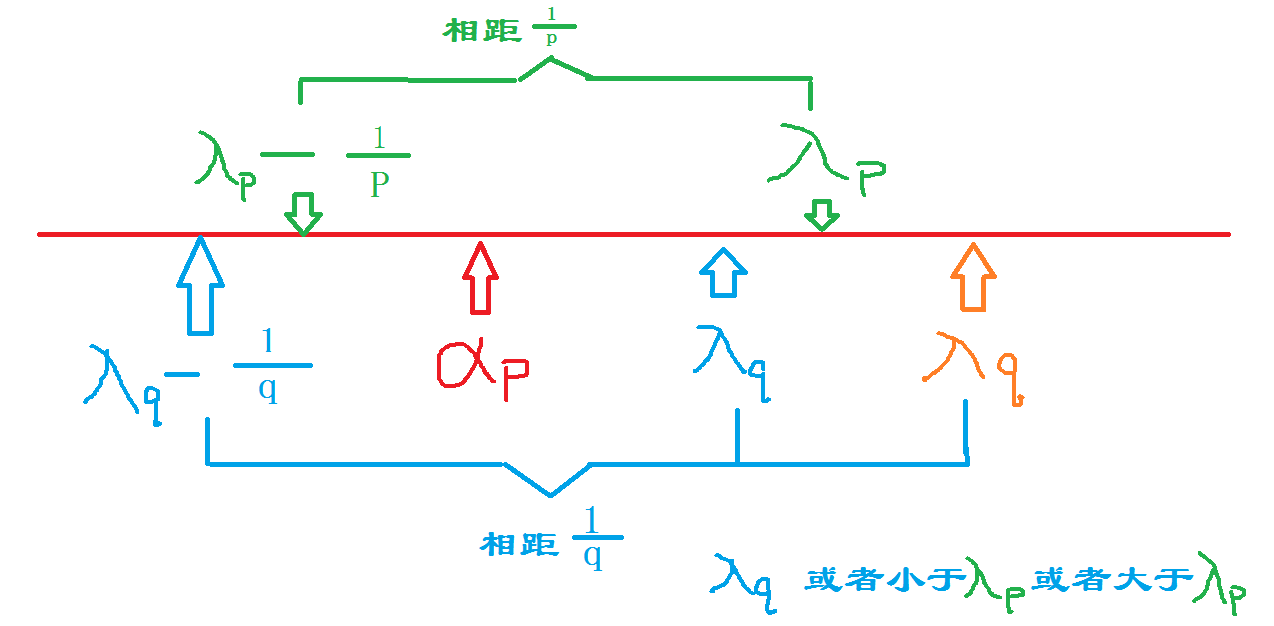# prove of supremum and infimum principle using Cauchy convergence criterium

prove: suppose S is a non-empty set having upperbound number set
by Archimedes property, for any positive number (alpha),exists a
integer,it makes (lambda_{alpha}alpha) a S's upperbound, while
(lambda) - a ,being not a upperbound to S.
so,
(lambda_{alpha}=k_{alpha}alpha) , a upperbound to S
(lambda_{alpha}-alpha=(k_{alpha}-1)alpha) , not a upperbound to S
set (alpha=frac{1}{n}),n=1,2,3....
so, for each positive n ,exists a corresponding (lambda_{n}),
it makes a upperbound to S, but not (lambda_{n}-frac{1}{n})
they are as belows:
(lambda_{alpha}=k_{alpha}alpha)
(lambda_{alpha}-alpha=(k_{alpha}-1)alpha)
so, there must be a (alpha'in S),with:
(alpha'>lambda_{n}-frac{1}{n})
so,get p(in {1,2,3,....})
(lambda_{p}=k_{p}frac{1}{p})
there must be a (alpha_{p})
(alpha_{p}>lambda_{p}-frac{1}{p})
choose q (in){1,2,3,...},we have:
(lambda_{q}=k_{q}frac{1}{q}) is an upperbound .
so (lambda_{q}>alpha_{p}>lambda_{p}-frac{1}{p})
so (lambda_{p}-lambda_{q}<frac{1}{p})
similarly,we get (lambda_{q}-lambda_{p}<frac{1}{q})so,we get |(lambda_{q}-lambda_{p}|<MAX{frac{1}{p},frac{1}{q}})
(forall epsilon>0),we choose N>(frac{1}{epsilon})
then we get (frac{1}{N}<epsilon)
so when p,q >N,then
(frac{1}{p},frac{1}{q}<frac{1}{N}<epsilon)
so |(lambda_{p}-lambda_{q}|<MAX{frac{1}{p},frac{1}{q}}<epsilon)
so , by Cauchy convergence criterium,({lambda_{n}}) converges
set (lim_{n oinfty}lambda_{n}=lambda)
\$forall alpha in S,and forall lambda_{n},alpha leqslant lambda_{n}
so ,by 数列极限的保不等式性， (forall alpha in S, alpha <lim_{n o infty}lambda_{n}=lambda)
so, (lambda) is a upperbound to S.
we need to prove :(forall epsilon>0),there exists a (alpha_{n}),with (alpha_{n}>lambda-epsilon)
we know (forall frac{1}{n},)there is a (alpha_{n}>lambda_{n}-frac{1}{n})
if (lambda_{n}-frac{1}{n}>lambda-epsilon)
ie, (lambda-lambda_{n}+frac{1}{n}<epsilon)
we need to have (frac{1}{n}<frac{epsilon}{2}),and (lambda-lambda_{n}<frac{epsilon}{2})
ie: (n>frac{2}{epsilon}),(lambda-lambda_{n}<frac{epsilon}{2})
cause (lim_{n oinfty}lambda_{n}=lambda),
so, (exists)N_{0}(in N^+),when n>(N_{0}),(|lambda-lambda_{n}|<frac{epsilon}{2})
ie,(-frac{epsilon}{2}<lambda-lambda_{n}<frac{epsilon}{2})
so, if n>MAX{(N_{0},frac{2}{epsilon}})
the theorem is proven
likely to prove the infimum

## 相关文章

### 函数作用域和匿名函数

'''作用域 作用域在定义函数时就已经固定住了，不会随着调用位置的改变而改变 ''' deftest1(): 　　print('in the test1') deftest2(): 　　print('in the test2') 　　returntest1 res =test2() print(res()) name = 'alex' de...